# Python Program to Remove an Element from a List

In this article, I've created some programs in Python that delete or remove an element (or multiple elements) from a list. The user must enter both elements and lists at runtime. Here is a list of programs:

## Remove an element from the list by value

This program removes an element (entered by the user) from a list (also entered by the user) by value. The question is: write a Python program to delete an element from a list. Here is its answer:

```print("Enter 10 Elements: ")
arr = []
for i in range(10):
arr.append(input())

print("\nEnter the Value to Delete: ")
val = input()

arr.remove(val)

print("\nThe New list is:")
print(arr)```

Here is its sample run: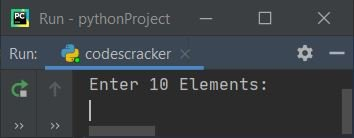Now supply the input "1, 2, 3, 4, 5, 6, 7, 8, 9, 10" as ten elements, and a value of "4" to delete it from the list and print the new list as shown in the snapshot given below: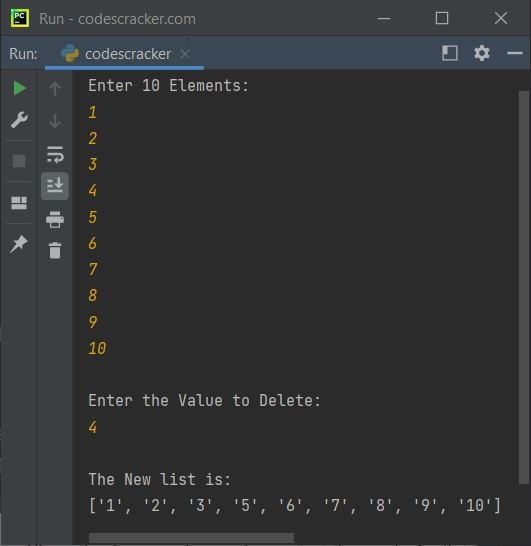Note: The "append()" method appends an element at the end of a list.

Note: The "remove()" method removes an element (by value provided as its argument) from a list.

In the above program, the following code:

`for i in range(10):`

is used to execute the following statement:

`arr.append(input())`

ten times with a value of "i" from 0 to 9. Therefore, all ten numbers entered by the user as provided in the sample run get stored in the list "arr[]" in this way:

`arr = ['1', '2', '3', '4', '5', '6', '7', '8', '9', '10']`

Now, using the following statement:

`arr.remove(val)`

The value stored in the "val" variable gets removed from the list "arr". For example, if the value of "val" is 4, then "4" gets deleted from the list named "arr".

### Delete Element from List of Given Size

This is a modified version of the previous program with an extra feature added to it. For example, this program allows the user to define the size of the list. The "end" used in this program is to skip inserting an automatic newline using "print()."

```print(end="Enter the Size of List: ")
tot = int(input())

arr = []
print(end="Enter " +str(tot)+ " Elements: ")
for i in range(tot):
arr.append(input())

print(end="\nEnter the Value to Delete: ")
val = input()

if val in arr:
arr.remove(val)
print("\nThe New list is: ")
for i in range(tot-1):
print(end=arr[i]+" ")
else:
print("\nElement doesn't exist in the List!")```

Here is its sample run with user input: "5" as the size of the list, "1, 2, 3, 4, 5" as the five elements of the list, and "4" as the element to delete: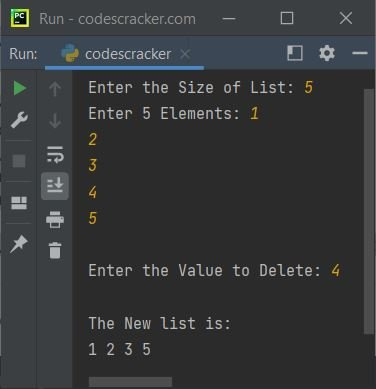Here is another sample run with user input "12" as the size of the list and "c, o, d, e, s, c, r, a, c, k, e, r" as the twelve elements of the list, then "c" as the element to delete from this list: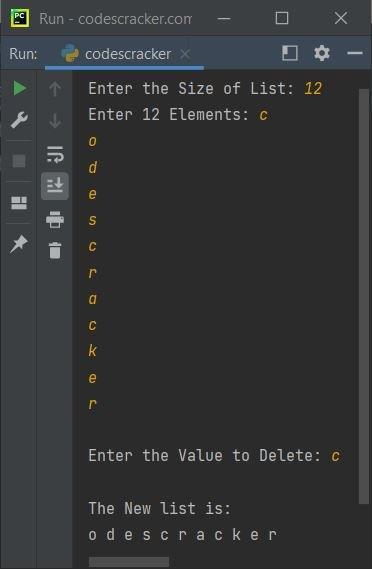Note: As you can see from this sample run, the first occurrence of "c" gets deleted from the list. But what if the user wants to delete the "c" at the second occurrence? Then we've got to follow the program that deletes an element from a list by index, as given below.

Note: The "str()" method converts any type of value to a string type.

## Remove an element from the list by index

The "pop()" method is used to delete an element from a list by index number. Whereas the "remove()" method is used to delete an element by its value, let's have a look at the program given below:

```print(end="Enter the Size of List: ")
tot = int(input())

arr = []
print(end="Enter " +str(tot)+ " Elements: ")
for i in range(tot):
arr.append(input())

print(end="\nEnter the Index Number: ")
index = int(input())

if index<tot:
arr.pop(index)
print("\nThe New list is: ")
for i in range(tot-1):
print(end=arr[i]+" ")
else:
print("\nInvalid Index Number!")```

Here is its sample run with user input: "12" as size and "c, o, d, e, s, c, r, a, c, k, e, r" as twelve elements of the list. To delete the second "c" from the list, enter "5" as the index number. Because the second 'c' is available at the fifth index, Indexing starts with 0.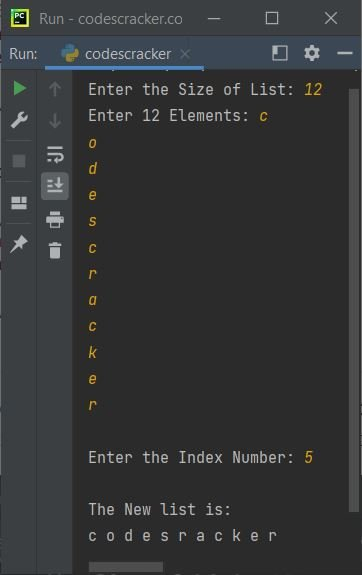The dry run of the above program with the same user input as provided in the sample run of this program goes like this:

• Initial values, tot = 12 (entered by user), arr = ['c', 'o', 'd', 'e', 's', 'c', 'r', 'a', 'c', 'k', 'e', 'r'] (also entered by user)
• Since indexing starts with 0. That is, the for loop through which the element of list arr gets received, starts with its i's value from 0 to 9, therefore all twelve elements of list stored in following ways:
• arr = 'c'
• arr = 'o'
• arr = 'd'
• arr = 'e'
• arr = 's'
• arr = 'c'
• and so on upto
• arr = 'r'
• Therefore to delete an element at 5th index, enter 5, that deletes second 'c' from the list
• When user enters 5 as index number to delete, then index=5
• Now the condition (of if) index<tot or 5<12 evaluates to be true, therefore program flow goes inside the if's body
• Inside if's body, the following statement:
`arr.pop(index)`
deletes the element from list, available at index number index or 5
• Now just print the new list as output

## Remove an element with all occurrences from the list

Now this program deletes all occurrences of an element by value. For example, if the user enters "1, 2, 3, 2, 4, 2" as six elements and "2" as an element to delete, then all three 2s get deleted from the list:

```print(end="Enter the Size of List: ")
tot = int(input())

arr = []
print(end="Enter " +str(tot)+ " Elements: ")
for i in range(tot):
arr.append(input())

print(end="\nEnter an Element to Delete with all Occurrences: ")
num = input()

if num in arr:
while num in arr:
arr.remove(num)

print("\nThe New list is: ")
tot = len(arr)
for i in range(tot):
print(end=arr[i] + " ")
else:
print("\nElement doesn't Found in the List!")```

Here is its sample run with user input "6" as size, "1, 2, 3, 2, 4, 2" as six elements, and "2" as an element to delete with all its occurrences: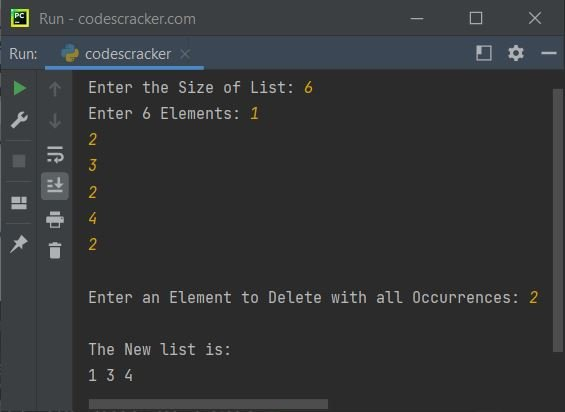In the above program, the following block of code:

```while num in arr:
arr.remove(num)```

states that the statement inside the "while", that is, "arr.remove(num)," gets executed until the condition "num in arr" evaluates to be false. The "num in arr" code checks whether the value of "num" is available in the list named "arr" or not. If available, then the condition evaluates to be true; otherwise, it evaluates to be false.

## Remove multiple elements from the list

This program allows the user to delete multiple elements from a list at one time. For example, if the given elements of a list are "1, 2, 3, 4, 5, 6, 7, 8," and if the user wants to delete two elements from the list, then enter 2 as the number of elements to delete, and then two values say "3" and "6" to delete these two elements, as shown in the program and its output given below:

```print(end="Enter the Size of List: ")
tot = int(input())

arr = []
print(end="Enter " +str(tot)+ " Elements: ")
for i in range(tot):
arr.append(input())

print(end="How many Elements to Delete ? ")
noOfElement = int(input())

print(end="Enter " +str(noOfElement)+ " Elements to Delete: ")

delList = []
for i in range(noOfElement):
delList.append(input())

for i in range(noOfElement):
if delList[i] in arr:
while delList[i] in arr:
arr.remove(delList[i])

print("\nThe New list is: ")
tot = len(arr)
for i in range(tot):
print(end=arr[i] + " ")```

Here is its sample run with the following user inputs:

• "6" as the size of the list
• "1, 2, 3, 2, 3, 4" as six elements of a list
• "2" is the number of elements to delete from the list
• "2, 3" as two elements to delete from the list

Let's have a look at the sample run given below: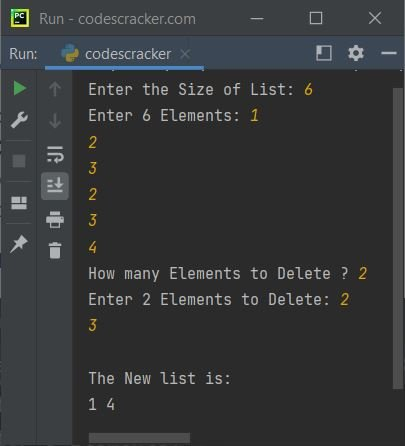Note: The "len()" returns the length of the thing (list) passed as its argument.

In the above program, I've created a list named "delList", that stores a list of elements (entered by the user) that have to be deleted from the list named "arr" (also entered by the user). That is, using the indexing of the "delList" list, I've checked whether the current element is available in the original list named "arr" or not. If available, then delete; otherwise, continue to check for next until the last element of "delList."

Python Online Test

« Previous Program Next Program »